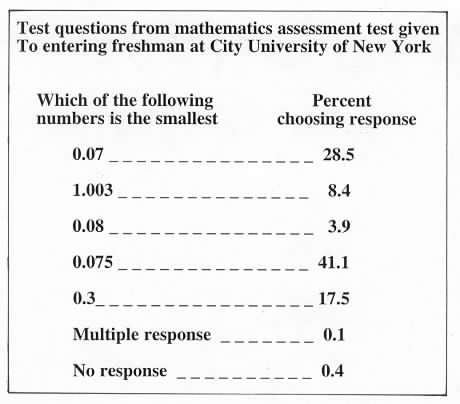Home
 Place Value .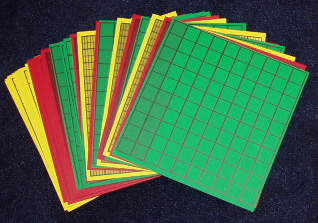Materials: Deck of Decimal Squares per group . Place the green transparency for .35 on the overhead and ask how its shaded amount can be described using decimals. Write some responses beneath the square. Two possibilities are shown on the left below. Repeat this activity for the yellow transparent square for .475. .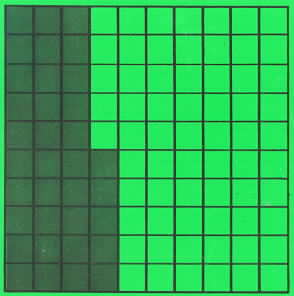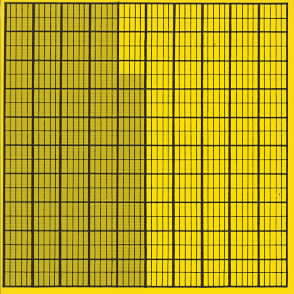.35 (thirty-five hundredths) .475 (475 thousandths) or or .3 (three tenths) and .05 (five hundredths) .4 (4 tenths) and .07 (7 hundredths) and .005 (5 thousandths) . Place Value TableHave each person select a yellow square and determine the number of tenths hundredths and thousandths. Sketch a place value table on the overhead and ask for volunteers to write in the digits for their decimals. A master sheet for place value tables is on the end pages of the Decimal Squares Teacher's Guide. Place Value Game Briefly explain the rules and illustrate one or two turns. Players will need a die and a sketch of a place value table or a copy of the Place Value Tables from the end pages of the Decimal Squares Teacher’s Guide. This game is on page 79 of the Decimal Squares Teacher’s Guide.These students are playing the Place Value Game with place value tables for five-digit mixed decimals. In turn each student rolls the die to obtain one of their digits.Photo courtesy of Herb Moyer Equality Write the equalities .3 = .30 = .300 on the overhead and ask for explanations as to why they are true. Someone may note that the squares for .3 and .300 have the same amount of shading. Others may state a rule for affixing zeros but be unable to explain why the rule holds. If .3 is written as 3/10 the numerator and denominator can be multiplied by 10 to obtain 30/100 which equals .30. .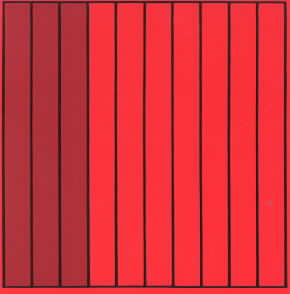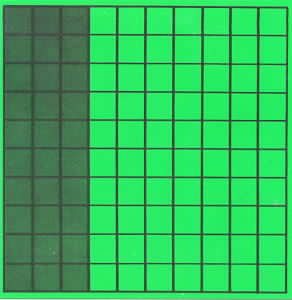.3 .30 .300 Or someone may explain these inequalities by using a place value table which will have a 3 in the tenths column and zeros in the hundredths and thousandths columns. Discuss the need for models to help make sense of equalities obtained from "placing a zero at the end of a decimal". . Inequality Write the inequalities .45 < .6 and .35 > .175 on the overhead and ask for explanations. One method of explaining these inequalities is to compare their squares. For example the square for .45 has between 4 and 5 full columns shaded and the square for .6 has 6 full columns shaded. .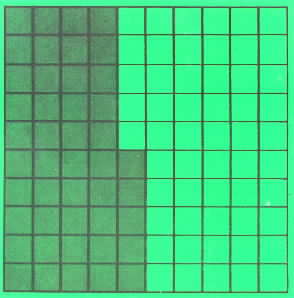Or a place value table will have a 4 in the tenths column for .45 and a 6 in the tenths column for .6. Discuss the need for models to teach an understanding of place value so that inequalities of decimals will make sense. Show a transparency of the following article by Ann Grossman "Decimal Notation: an Important Research Finding" from NCTM’s Arithmetic Teacher. Discuss plausible reasons for the high percentage of incorrect selections. For example some students believe that the more decimal places in a number the smaller the number.